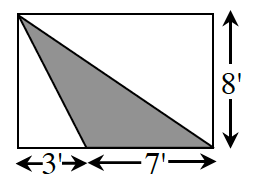### Home > CCG > Chapter 2 > Lesson 2.2.2 > Problem2-75

2-75.

The shaded triangle below is surrounded by a rectangle. Find the area of the triangle.The formula to find the area of a triangle is $\frac{1}{2}(\text{base}\times\text{height})$.

$28$ square feet.